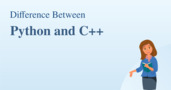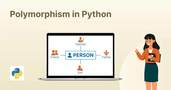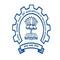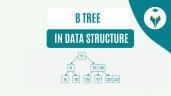Python Program to Swap Two Variables

# Python Program to Swap Two Variables

clickHere
Updated on Jan 19, 2023 14:42 IST

In this article, we will learn how to swap two variables using the Python programming language. Here’s the agenda!

Python Program to Swap Two Variables

There are multiple ways to do this. So, Swapping 2 variables in python simply means swapping their values!

Explore- Python Online Courses & Certifications

For example consider 2 variables a and b and their values to be 10 and 20. So using python, we can swap the values of a and b to be 20 and 10. So without further delay, let us jump into examples and see what are the different ways in which you can swap 2 variables using python.

## Program 1: Using Arithmetic Operators

We can make use of arithmetic operators in python to swap 2 variables

• Using the addition and subtraction operator

The goal is to find the sum in one of the two numbers provided. The sum and subtraction from the sum can then be used to swap the integers.

• Using multiplication and division operator

The goal is to multiply the two integers that are supplied. The division can then be used to calculate the numbers.

` `
`#declaring 2 variablesi = 3j = 4#swapping the values of 2 variables using addition and subtraction operatori = i+jj = i-ji = i-j#printing values after swappingprint("value of i after swapping : ",i)print("value of j after swapping : ",j)#declaring 2 variablesc = 5d = 6#swapping the values of 2 variables using multiplication and division operatorc = c*dd = c/dc = c/d#printing values after swappingprint("value of c after swapping : ",c)print("value of d after swapping : ",d)Copy code`

Output:

## Program 2: Using Bitwise addition and subtraction for swapping

Here we use Bitwise operation of addition and subtraction for swapping 2 variables

` `
`#declaring 2 variablese = 8f = 9#swapping the values of 2 variables Using Bitwise addition and subtraction for swappinge = (e & f) + (e | f)f = e + (~f) + 1e = e + (~f) + 1#printing values after swappingprint("value of e after swapping : ",e)print("value of f after swapping : ",f)Copy code`

Output:

## Program 3: Using XOR Operator

To swap two variables, you can also use the bitwise XOR operator. When you perform an XOR operation on two integers x and y, the result is a number with all bits set to 1 wherever the bits of x and y differ.

` `
`#declaring 2 variablesy = 1z = 2#swapping the values of 2 variables using XOR operatory = y^zz = y^zy = y^z#printing values after swappingprint("value of y after swapping : ",y)print("value of z after swapping : ",z)Copy code`

Output:

## Program 4: Using Comma Operator / without using the temporary variable

` `
`#declaring 2 variablesh = 30v = 60#swapping the values of 2 variables using comma operatorh,v = v,h#printing values after swappingprint("value of h after swapping : ",h)print("value of v after swapping : ",v)Copy code`

Output:The Difference Between C and Python
C and Python are two of the most popular programming languages used by developers these days. C has been in existence for many years now, whereas Python has come into...read morePython vs. C++ – What’s the Difference?
Python and C++ both are general-purpose programming languages. However, their usage and syntax differ widely. In this article, we will highlight the most prominent differences between the two languages.In Python, polymorphism means the same function name is being used for different types. In this article, we will learn about polymorphism and how to use it with inheritance.

## Program 5: Using Temporary Variable

The simplest way is to store the value of one variable (say x) in a temporary variable and then assign the value of variable y to the variable x. Finally, assign the value of the temporary variable to the variable y.

` `
`#declaring 2 variablesa = 20d = 70#swapping the values of 2 variables using temp variabletemp = aa = dd = temp#printing values after swappingprint("value of a after swapping : ",a)print("value of d after swapping : ",d)Copy code`

Output:

## Conclusion

clickHere

This is a collection of insightful articles from domain experts in the fields of Cloud Computing, DevOps, AWS, Data Science, Machine Learning, AI, and Natural Language Processing. The range of topics caters to upski... Read Full Bio

## Trending Technology CoursesPython Training
IIT Bombay4.2Python Fundamentals for Beginners
Great Learning4.5Programming for Everybody (Getting Started with Python)Programming for Everybody (Getting Started with Py...
Coursera4.5Python Fundamentals for Beginners
Great Learning4.5Starts 11 JanData/Web scraping with Python
Great Learning4.0Starts 11 JanAnalytics With SQL And Python
Great Learning4.5Starts 11 Jan

## Top Picks & New Arrivals